## Internal Class Diagram

60
0
1
publish time: 2020-09-15Kiraaaa

One additional benefit that composite structure diagrams have over class diagrams is their ability to show both internal relationships and external collaboration relationships between elements.

See More Related TemplatesThis is an example of a UML diagram about adding events to the calendar.
UML Diagram - Add event to calendar
23
1
0easy diagrams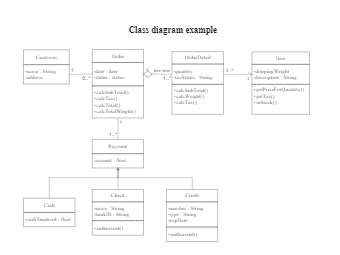This is an example of a uml class diagram.
Class diagram example
206
6
4easy diagrams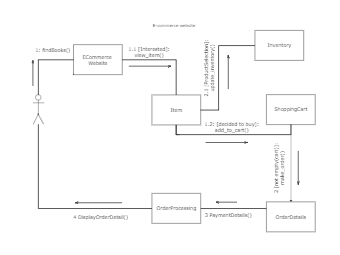This is an example of a UML collaboration diagram.
ecommerce website - UML diagram
25
2
0easy diagrams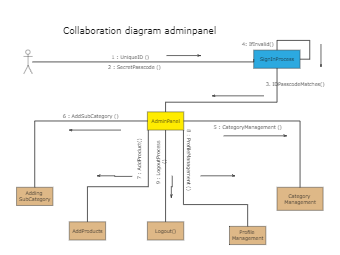11
1
0easy diagrams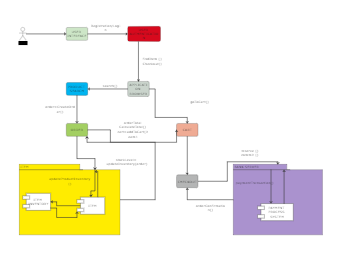This is an example of a UML communication diagram.
Communication diagram example
11
1
1easy diagrams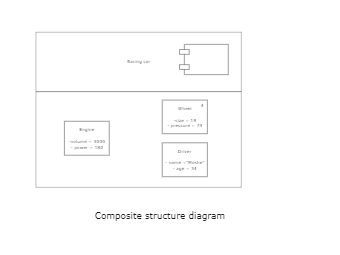This is an example of a composite structure diagram.
Composite structure diagram
3
0
0easy diagrams